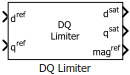# DQ Limiter

Saturate voltages (or current) in the dq reference frame

Since R2020a

•Libraries:
Motor Control Blockset / Controls / Control Reference
Motor Control Blockset HDL Support / Controls / Control Reference

## Description

The DQ Limiter block generates saturated values of the input voltages (or current) in the dq reference frame, depending on the specified saturation limit and the selected method of saturation.

The block accepts reference values of d and q axis voltages (or current) and outputs the corresponding saturated values. The block also provides the unsaturated peak value of the reference dq voltages (or current), which you can use to enable field weakening control.

Note

The block supports a fixed-point data type size up to 128 bits.

### Equations

These equations describe the computation of saturated dq voltage (or current) values by the block.

For DQ equivalence:

`$ma{g}^{ref}=\sqrt{{\left({d}^{ref}\right)}^{2}+{\left({q}^{ref}\right)}^{2}}$`

When $ma{g}^{ref}>{x}_{\mathrm{max}}$.

• ${d}^{sat}=\frac{{d}^{ref}}{ma{g}^{ref}}×{x}_{\mathrm{max}}$

• ${q}^{sat}=\frac{{q}^{ref}}{ma{g}^{ref}}×{x}_{\mathrm{max}}$

When $ma{g}^{ref}\le {x}_{\mathrm{max}}$.

• ${d}^{sat}={d}^{ref}$

• ${q}^{sat}={q}^{ref}$

Where, xmax is the saturation limit.

When D-axis is prioritized:

`$ma{g}^{ref}=\sqrt{{\left({d}^{ref}\right)}^{2}+{\left({q}^{ref}\right)}^{2}}$`
`$\begin{array}{l}{d}^{sat}=\mathrm{min}\left(\mathrm{max}\left({d}^{ref},-{x}_{\mathrm{max}}\right),{x}_{\mathrm{max}}\right)\\ {q}^{sat}=sign\left({q}^{ref}\right)×\mathrm{min}\left(|{q}^{ref}|,|\sqrt{{x}_{\mathrm{max}}^{2}-{\left({d}^{sat}\right)}^{2}}|\right)\end{array}$`

Where, xmax is the saturation limit.

When Q-axis is prioritized:

`$ma{g}^{ref}=\sqrt{{\left({d}^{ref}\right)}^{2}+{\left({q}^{ref}\right)}^{2}}$`
`$\begin{array}{l}{q}^{sat}=\mathrm{min}\left(\mathrm{max}\left({q}^{ref},-{x}_{\mathrm{max}}\right),{x}_{\mathrm{max}}\right)\\ {d}^{sat}=sign\left({d}^{ref}\right)×\mathrm{min}\left(|{d}^{ref}|,|\sqrt{{x}_{\mathrm{max}}^{2}-{\left({q}^{sat}\right)}^{2}}|\right)\end{array}$`

Where, xmax is the saturation limit.

## Ports

### Input

expand all

Reference voltage (or current) value along the d-axis of the rotating dq reference frame.

Data Types: `single` | `double` | `fixed point`

Reference voltage (or current) value along the q-axis of the rotating dq reference frame.

Data Types: `single` | `double` | `fixed point`

Saturation method that the block uses to initiate saturation. The port accepts one of the following integer values:

• 1 – Prioritize D-axis

• 2 – Prioritize Q-axis

• Any other positive integer – D-Q equivalence method

#### Dependencies

To enable this port, select `Input port` for the Saturation method parameter.

Data Types: `uint16`

Saturation limit value that the block uses to initiate saturation. The port accepts values greater than or equal to 0.

#### Dependencies

To enable this port, select `Input port` for the Saturation limit parameter.

Data Types: `single` | `double` | `fixed point`

### Output

expand all

Saturated voltage (or current) value along the d-axis of the rotating dq reference frame.

Data Types: `single` | `double` | `fixed point`

Saturated voltage (or current) value along the q-axis of the rotating dq reference frame.

Data Types: `single` | `double` | `fixed point`

Unsaturated peak value of the input voltages (or current).

Data Types: `single` | `double` | `fixed point`

## Parameters

expand all

Select whether the block should use dialog box or input port to specify the saturated method.

#### Programmatic Use

`satMethodInputType`

Select the saturation method that the block should use.

#### Dependencies

To enable this parameter, set Saturation method to `Specify via dialog`.

#### Programmatic Use

`satMethodSelected`

Select whether the block should use dialog box or input port to specify the saturated limit.

#### Programmatic Use

`satLimitInputType`

The maximum magnitude of voltage (or current) above which the block outputs are limited.

is usually the rated current of the motor. When you work with the Per-Unit system (PU), you should convert the rated current of the motor to Per-Unit value with respect to the base current.

is usually the maximum phase voltage supplied by the inverter. Generally it is $\frac{{V}_{\text{dc}}}{\sqrt{3}}$ for Space Vector PWM and $\frac{{V}_{\text{dc}}}{2}$ for Sinusoidal PWM, where Vdc is the DC link voltage of the inverter.

Note

You can enter either per unit or SI unit voltage (or current) value in this parameter (unit of the entered value should be same as that of the dref and qref inputs). For optimum performance, we recommend that you provide a per unit value.

#### Dependencies

To enable this parameter, set Saturation limit to `Specify via dialog`.

`Vmax`

## Version History

Introduced in R2020a